# How to Find the Asymptotes of a Hyperbola

## Hyperbola

The hyperbola is a conic section. The term hyperbola is referred to the two disconnected curves shown in the figure.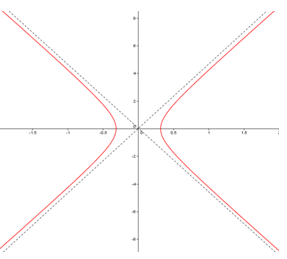If the principal axes are coinciding with the Cartesian axes, the general equation of hyperbola is of the form: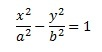These hyperbolas are symmetric around y axis and are known as y-axis hyperbola. The hyperbola symmetric around x-axis (or x-axis hyperbola) are given by the equation,

##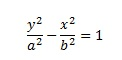How to find the asymptotes of a hyperbola

To find the asymptotes of a hyperbola, use a simple manipulation of the equation of the parabola.

i. First bring the equation of the parabola to above given form

If the parabola is given as mx2+ny2=l, by defining

a=√(l/m) and b=√(-l/n) where l<0

(This step is not necessary if the equation is given in standard from.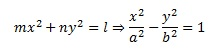ii. Then, replace the right hand side of the equation with zero.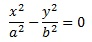iii. Factorize the equation and take solutions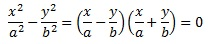Therefore, the solutions are ,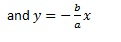Equations of the asymptotes are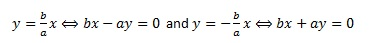Equations of the asymptotes for the x-axis hyperbola also can be obtained by the same procedure.

## Find the asymptotes of a hyperbola – Example 1

Consider the hyperbola given by the equation x2/4-y2/9=1. Find the equations of the asymptotes.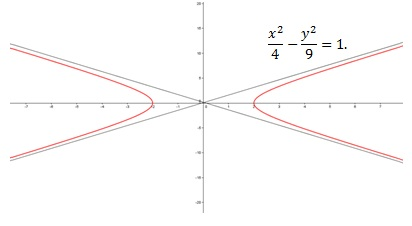Rewrite the equation and follow the above procedure.
x2/4-y2/9=x2/22 -y2/32 =1

By replacing the right hand side with zero, the equation becomes x2/22 -y2/32 =0.
Factorizing and taking solution of the equation give,

(x/2-y/3)(x/2+y/3)=0

Equations of the asymptotes are,

3x-2y=0 and 3x+2y=0

## Find the asymptotes of a hyperbola – Example 2

• Equation of a parabola is given as -4x² + y² = 4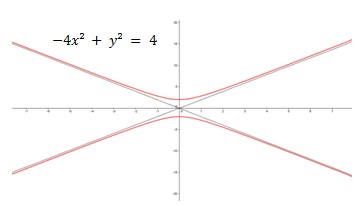This hyperbola is an x-axis hyperbola.
Rearranging the terms of the hyperbola into the standard from gives
-4x2+ y2= 4=>y2/22 -x2/12 =1
Factorizing the equation provides the following
(y/2-x)(y/2+x)=0
Therefore, the solutions are y-2x=0 and y+2x=0.